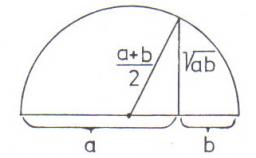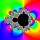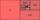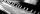# Geometric mean

Calculate the geometric mean of numbers a=15.2 and b=25.6. Determine the mean by construction where a and b are the length of the lines.

Result

m =  19.726

#### Solution:

$a=15.2 \ \\ b=25.6 \ \\ m=\sqrt{ a \cdot \ b }=\sqrt{ 15.2 \cdot \ 25.6 } \doteq 19.7261 \doteq 19.726$Our examples were largely sent or created by pupils and students themselves. Therefore, we would be pleased if you could send us any errors you found, spelling mistakes, or rephasing the example. Thank you!

Leave us a comment of this math problem and its solution (i.e. if it is still somewhat unclear...):Be the first to comment!Tips to related online calculators
Looking for a statistical calculator?

## Next similar math problems:

1. Geometric progression 2There is geometric sequence with a1=5.7 and quotient q=-2.5. Calculate a17.
2. Tenth memberCalculate the tenth member of geometric sequence when given: a1=1/2 and q=2
3. Geometric progressionFill 4 numbers between 4 and -12500 to form geometric progression.
4. CoefficientDetermine the coefficient of this sequence: 7.2; 2.4; 0.8
5. Gp - 80Sum of the first four members of a geometric progression is 80. Determine they if we know that the fourth member is nine times greater than the second.
6. Six termsFind the first six terms of the sequence a1 = -3, an = 2 * an-1
7. Geometric progression 48,4√2,4,2√2
8. PianoIf Suzan practicing 10 minutes at Monday; every other day she wants to practice 2 times as much as the previous day, how many hours and minutes will have to practice on Friday?
9. AverageIf the average(arithmetic mean) of three numbers x,y,z is 50. What is the average of there numbers (3x +10), (3y +10), (3z+10) ?
10. ProductThe product of two consecutive odd numbers is 8463. What are this numbers?
11. CalculationHow much is sum of square root of six and the square root of 225?
12. Theorem proveWe want to prove the sentence: If the natural number n is divisible by six, then n is divisible by three. From what assumption we started?
13. PowerNumber ?. Find the value of x.
14. ExaminationThe class is 21 students. How many ways can choose two to examination?Solve quadratic equation: 2x2-58x+396=0Write the first 7 members of an arithmetic sequence: a1=-3, d=6.Solve system of linear equations by elimination method: 5/2x + 3/5y= 4/15 1/2x + 2/5y= 2/15